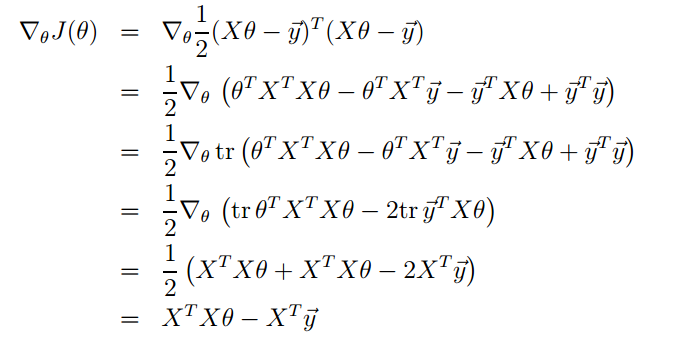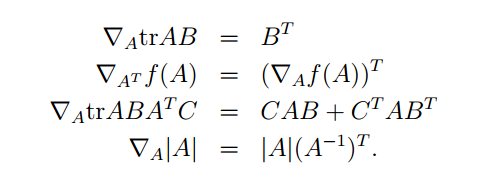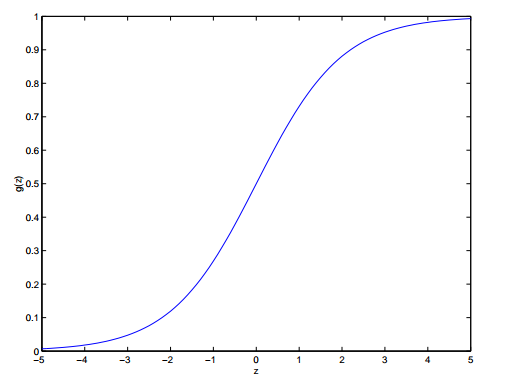## 最小二乘法

### 正则方程## logistic回归

### logistic函数

logistic函数$\sigma(x) = \frac{1}{1+e^{-x}}$，又叫sigmoid函数，将输入$(-\infty, +\infty)$压缩到$(0, 1)$之间。它的形状如下：### logistic回归

logistic函数的输出既然是在$(0,1)$上，我们可以将其作为概率。也就是说，我们认为它的输出是样本点属于类别$1$的概率：